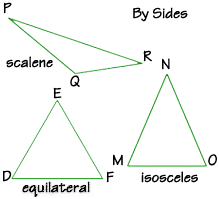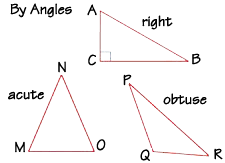Home    |    Teacher    |    Parents    |    Glossary    |    About UsJust as the rectangle and the circle are very popular in the real world, so is the triangle! You'll find triangles at work bracing a structure or bridge, racking billiard balls, or holding up a shelf. Triangles are classified in two general ways: by their sides and by their angles. First, we'll classify by sides:A triangle with three sides of different lengths is called a scalene triangle. An isosceles triangle has just two equal sides, called legs. The third side is called the base. The angles that are opposite the equal sides are also equal. An equilateral triangle has three equal sides. In this type of triangle, the angles are also equal, so it can also be called an equiangular triangle. Each angle of an equilateral triangle must measure 60 degrees, since the sum of the interior angles of any triangle must equal 180 degrees.Now let's classify by angles. An acute triangle has three acute angles, or three angles with a measure of less than 90 degrees. An obtuse triangle has one angle that is greater than 90 degrees. If one of the angles in a triangle is a right angle, then the triangle is called a right triangle. Notice we draw a square at vertex C to show a right angle.

You can use two labels for a triangle. For example, triangle MNO is both an acute and an isosceles triangle. Triangle PQR is an obtuse, scalene triangle.

 Homework Help | Geometry | PolygonsEmail this page to a friendSearch·  Polygon basics·  Triangles·  Quadrilaterals·  Area of polygons     and circlesFirst Glance In Depth Examples WorkoutTriangles